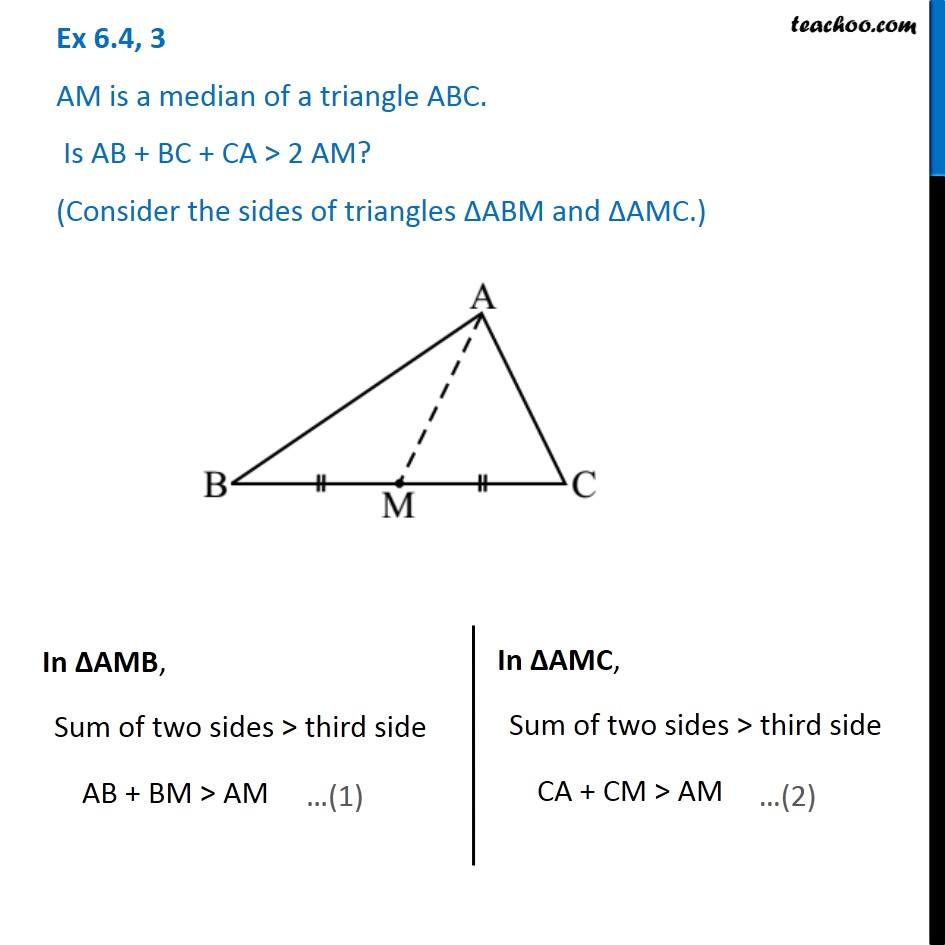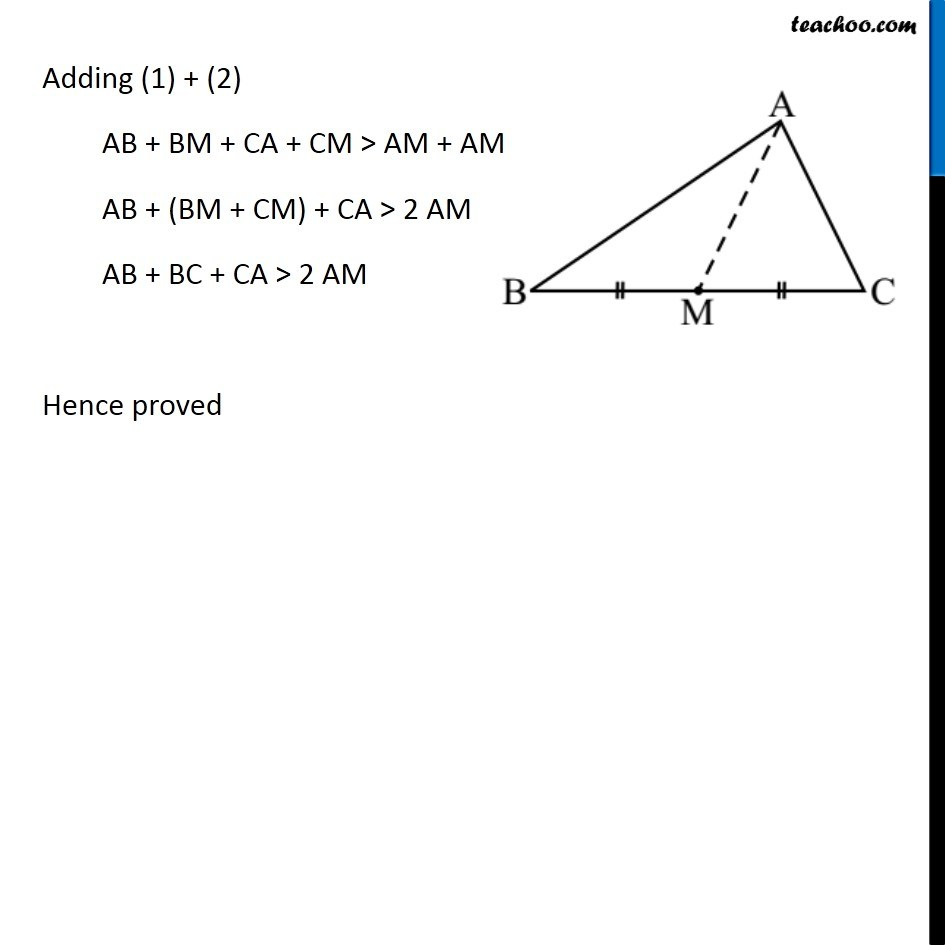1. Chapter 6 Class 7 Triangle and its Properties
2. Concept wise
3. Sum of lengths of two sides of a triangle

Transcript

Ex 6.4, 3 AM is a median of a triangle ABC. Is AB + BC + CA > 2 AM? (Consider the sides of triangles ∆ABM and ∆AMC.)In ∆AMB, Sum of two sides > third side AB + BM > AM In ∆AMC, Sum of two sides > third side CA + CM > AM Adding (1) + (2) AB + BM + CA + CM > AM + AM AB + (BM + CM) + CA > 2 AM AB + BC + CA > 2 AM Hence proved

Sum of lengths of two sides of a triangle